Anúncio

# Intro-QM-Chem.ppt

sami97008
29 de Mar de 2023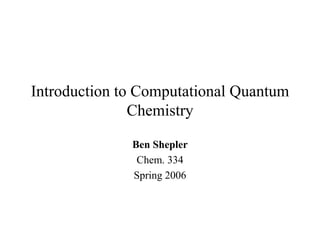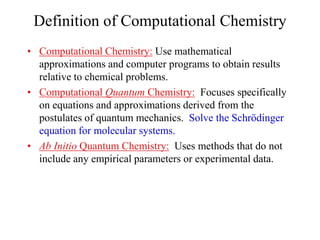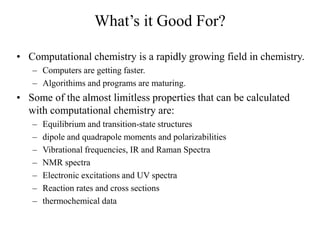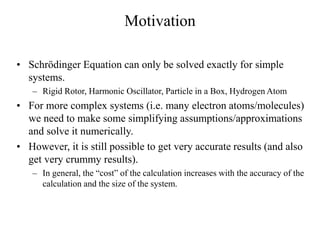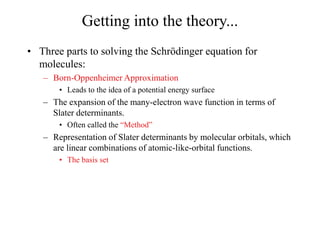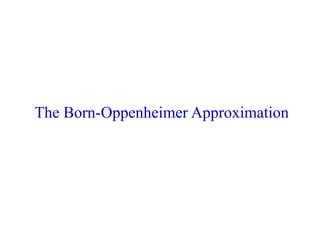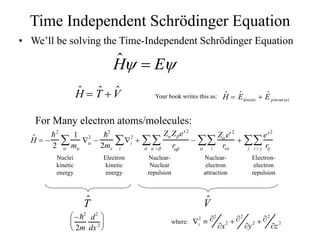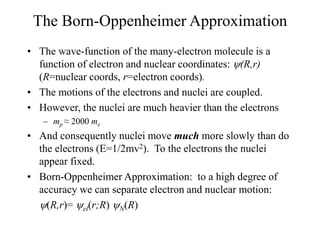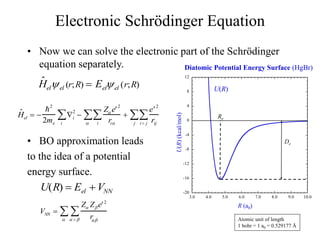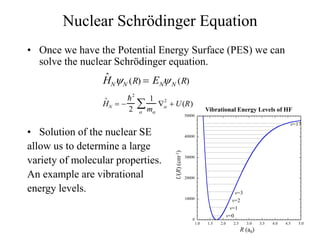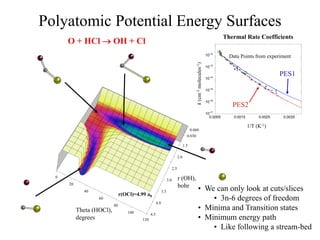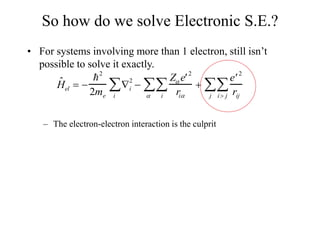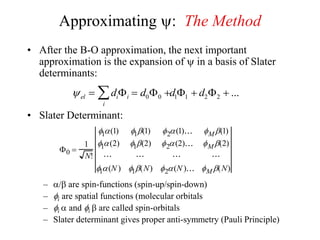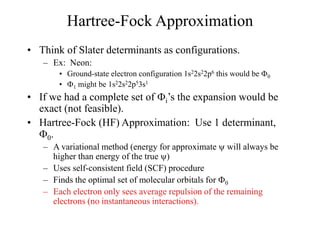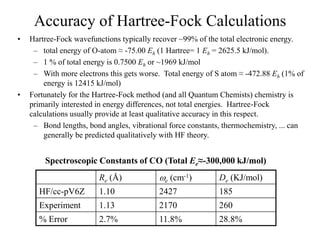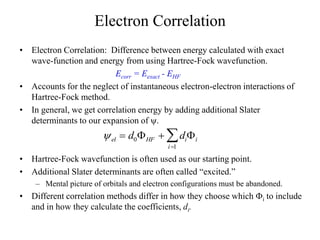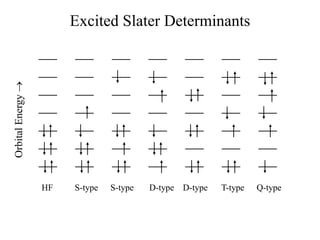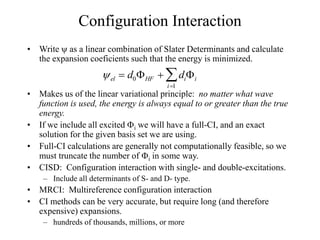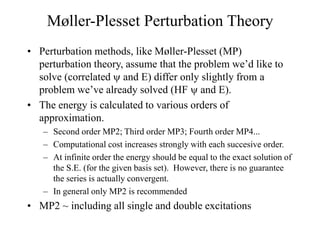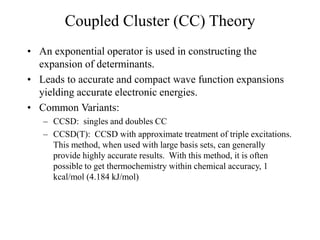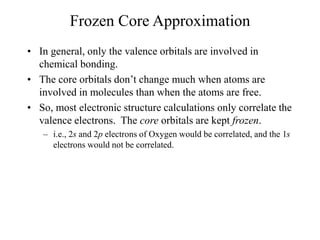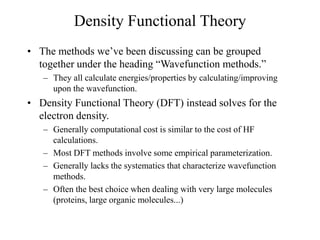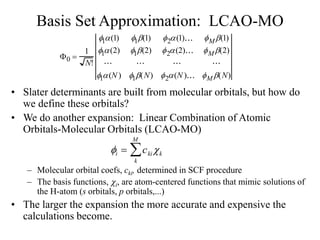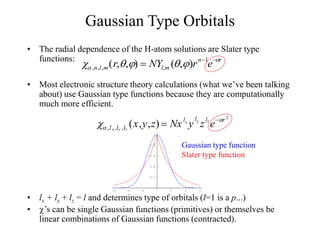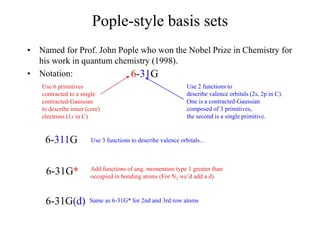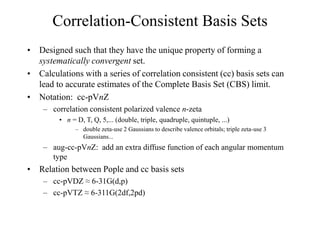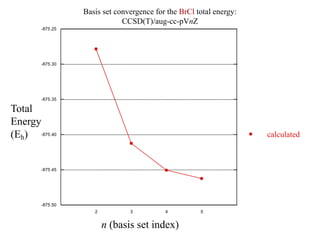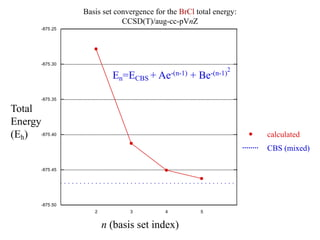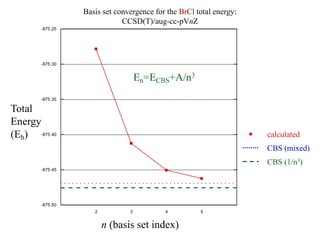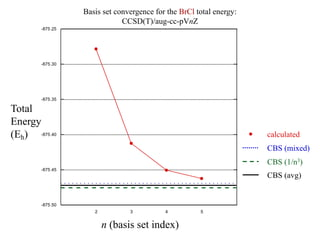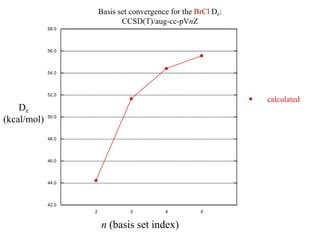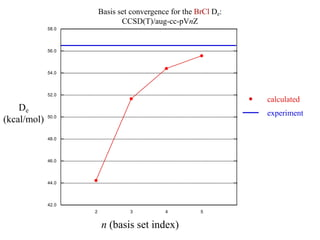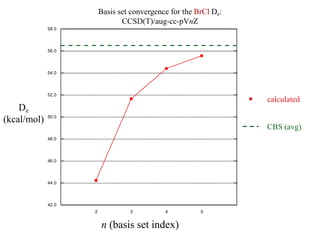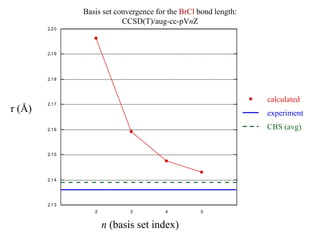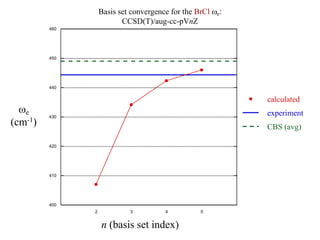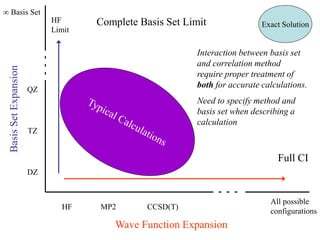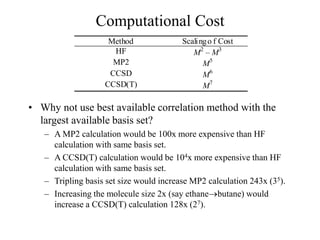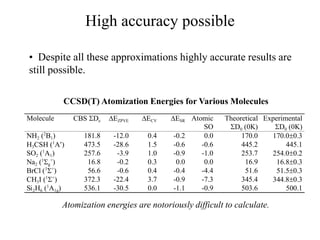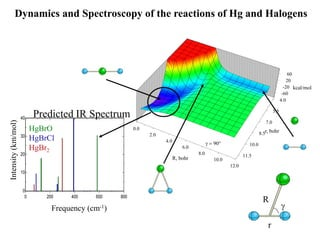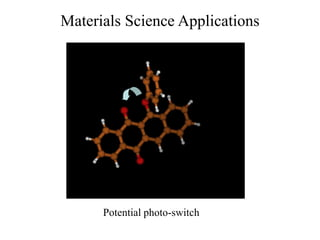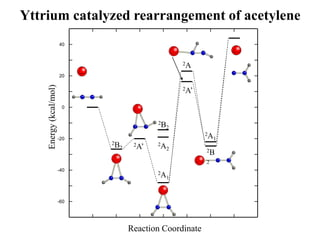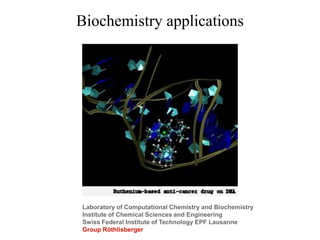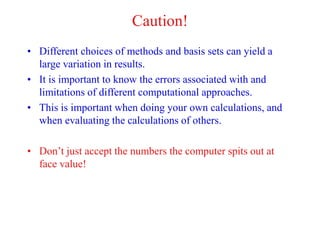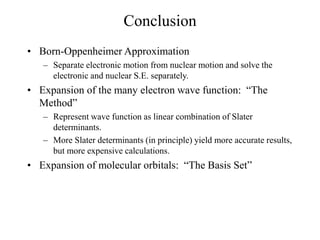1 de 47
Anúncio

### Intro-QM-Chem.ppt

1. Introduction to Computational Quantum Chemistry Ben Shepler Chem. 334 Spring 2006
2. Definition of Computational Chemistry • Computational Chemistry: Use mathematical approximations and computer programs to obtain results relative to chemical problems. • Computational Quantum Chemistry: Focuses specifically on equations and approximations derived from the postulates of quantum mechanics. Solve the Schrödinger equation for molecular systems. • Ab Initio Quantum Chemistry: Uses methods that do not include any empirical parameters or experimental data.
3. What’s it Good For? • Computational chemistry is a rapidly growing field in chemistry. – Computers are getting faster. – Algorithims and programs are maturing. • Some of the almost limitless properties that can be calculated with computational chemistry are: – Equilibrium and transition-state structures – dipole and quadrapole moments and polarizabilities – Vibrational frequencies, IR and Raman Spectra – NMR spectra – Electronic excitations and UV spectra – Reaction rates and cross sections – thermochemical data
4. Motivation • Schrödinger Equation can only be solved exactly for simple systems. – Rigid Rotor, Harmonic Oscillator, Particle in a Box, Hydrogen Atom • For more complex systems (i.e. many electron atoms/molecules) we need to make some simplifying assumptions/approximations and solve it numerically. • However, it is still possible to get very accurate results (and also get very crummy results). – In general, the “cost” of the calculation increases with the accuracy of the calculation and the size of the system.
5. Getting into the theory... • Three parts to solving the Schrödinger equation for molecules: – Born-Oppenheimer Approximation • Leads to the idea of a potential energy surface – The expansion of the many-electron wave function in terms of Slater determinants. • Often called the “Method” – Representation of Slater determinants by molecular orbitals, which are linear combinations of atomic-like-orbital functions. • The basis set
6. The Born-Oppenheimer Approximation
7. Time Independent Schrödinger Equation • We’ll be solving the Time-Independent Schrödinger Equation ˆ H  E ˆ H  ˆ T  ˆ V ˆ H  ˆ Ekinetic  ˆ Epot ent ial Your book writes this as: ˆ H   2 2 1 m  2    2 2me i 2 i   Z Z  e 2 r        Z  e 2 ri i      e 2 rij i  j  j  For Many electron atoms/molecules: Nuclei kinetic energy Electron kinetic energy Nuclear- Nuclear repulsion Nuclear- electron attraction Electron- electron repulsion ˆ T ˆ V i 2  2 x2  2 y2  2 z2 where:  2 2m d2 dx2      
8. The Born-Oppenheimer Approximation • The wave-function of the many-electron molecule is a function of electron and nuclear coordinates: (R,r) (R=nuclear coords, r=electron coords). • The motions of the electrons and nuclei are coupled. • However, the nuclei are much heavier than the electrons – mp ≈ 2000 me • And consequently nuclei move much more slowly than do the electrons (E=1/2mv2). To the electrons the nuclei appear fixed. • Born-Oppenheimer Approximation: to a high degree of accuracy we can separate electron and nuclear motion: (R,r)= el(r;R) N(R)
9. -20 -16 -12 -8 -4 0 4 8 12 3.0 4.0 5.0 6.0 7.0 8.0 9.0 10.0 Electronic Schrödinger Equation • Now we can solve the electronic part of the Schrödinger equation separately. • BO approximation leads to the idea of a potential energy surface. Diatomic Potential Energy Surface (HgBr) U(R) (kcal/mol) R (a0) Atomic unit of length 1 bohr = 1 a0 = 0.529177 Å U(R) De Re ˆ Helel (r;R)  Eelel (r;R) ˆ Hel   2 2me i 2 i   Z  e 2 ri i      e 2 rij i j  j  U(R)  Eel  VNN VNN  Z Z  e 2 r       
10. Nuclear Schrödinger Equation • Once we have the Potential Energy Surface (PES) we can solve the nuclear Schrödinger equation. • Solution of the nuclear SE allow us to determine a large variety of molecular properties. An example are vibrational energy levels. ˆ HNN (R)  ENN (R) ˆ HN   2 2 1 m  2    U(R) 0 10000 20000 30000 40000 50000 1.0 1.5 2.0 2.5 3.0 3.5 4.0 4.5 5.0 Vibrational Energy Levels of HF U(R) (cm -1 ) R (a0) v=0 v=1 v=2 v=3 v=17
11. Polyatomic Potential Energy Surfaces 120 100 80 60 40 20 0 1.5 2.0 2.5 3.0 3.5 4.0 4.5 0.030 0.060 Theta (HOCl), degrees r (OH), bohr r(OCl)=4.99 a0 10-17 10-16 10-15 10-14 10-13 10-12 0.0005 0.0015 0.0025 0.0035 Thermal Rate Coefficients PES1 Data Points from experiment PES2 1/T (K-1) k (cm -3 molecules -1 ) • We can only look at cuts/slices • 3n-6 degrees of freedom • Minima and Transition states • Minimum energy path • Like following a stream-bed O + HCl  OH + Cl
12. The Method
13. So how do we solve Electronic S.E.? • For systems involving more than 1 electron, still isn’t possible to solve it exactly. – The electron-electron interaction is the culprit ˆ Hel   2 2me i 2 i   Z  e 2 ri i      e 2 rij i j  j 
14. Approximating : The Method • After the B-O approximation, the next important approximation is the expansion of  in a basis of Slater determinants: • Slater Determinant: – / are spin-functions (spin-up/spin-down) – i are spatial functions (molecular orbitals – i  and i  are called spin-orbitals – Slater determinant gives proper anti-symmetry (Pauli Principle) el  dii  d00  i  d11  d22  ... 0  1 N! 1(1) 1(1) 2(1) M(1) 1(2) 1(2) 2(2) M(2) 1(N ) 1(N) 2(N ) M (N)
15. Hartree-Fock Approximation • Think of Slater determinants as configurations. – Ex: Neon: • Ground-state electron configuration 1s22s22p6 this would be 0 • 1 might be 1s22s22p53s1 • If we had a complete set of i’s the expansion would be exact (not feasible). • Hartree-Fock (HF) Approximation: Use 1 determinant, 0. – A variational method (energy for approximate  will always be higher than energy of the true ) – Uses self-consistent field (SCF) procedure – Finds the optimal set of molecular orbitals for 0 – Each electron only sees average repulsion of the remaining electrons (no instantaneous interactions).
16. Accuracy of Hartree-Fock Calculations • Hartree-Fock wavefunctions typically recover ~99% of the total electronic energy. – total energy of O-atom ≈ -75.00 Eh (1 Hartree= 1 Eh = 2625.5 kJ/mol). – 1 % of total energy is 0.7500 Eh or ~1969 kJ/mol – With more electrons this gets worse. Total energy of S atom ≈ -472.88 Eh (1% of energy is 12415 kJ/mol) • Fortunately for the Hartree-Fock method (and all Quantum Chemists) chemistry is primarily interested in energy differences, not total energies. Hartree-Fock calculations usually provide at least qualitative accuracy in this respect. – Bond lengths, bond angles, vibrational force constants, thermochemistry, ... can generally be predicted qualitatively with HF theory. Re (Å) e (cm-1) De (KJ/mol) HF/cc-pV6Z 1.10 2427 185 Experiment 1.13 2170 260 % Error 2.7% 11.8% 28.8% Spectroscopic Constants of CO (Total Ee≈-300,000 kJ/mol)
17. Electron Correlation • Electron Correlation: Difference between energy calculated with exact wave-function and energy from using Hartree-Fock wavefunction. Ecorr = Eexact - EHF • Accounts for the neglect of instantaneous electron-electron interactions of Hartree-Fock method. • In general, we get correlation energy by adding additional Slater determinants to our expansion of . • Hartree-Fock wavefunction is often used as our starting point. • Additional Slater determinants are often called “excited.” – Mental picture of orbitals and electron configurations must be abandoned. • Different correlation methods differ in how they choose which i to include and in how they calculate the coefficients, di. el  d0HF  dii i1 
18. Excited Slater Determinants Orbital Energy  HF S-type S-type D-type D-type T-type Q-type
19. Configuration Interaction • Write  as a linear combination of Slater Determinants and calculate the expansion coeficients such that the energy is minimized. • Makes us of the linear variational principle: no matter what wave function is used, the energy is always equal to or greater than the true energy. • If we include all excited i we will have a full-CI, and an exact solution for the given basis set we are using. • Full-CI calculations are generally not computationally feasible, so we must truncate the number of i in some way. • CISD: Configuration interaction with single- and double-excitations. – Include all determinants of S- and D- type. • MRCI: Multireference configuration interaction • CI methods can be very accurate, but require long (and therefore expensive) expansions. – hundreds of thousands, millions, or more el  d0HF  dii i1 
20. Møller-Plesset Perturbation Theory • Perturbation methods, like Møller-Plesset (MP) perturbation theory, assume that the problem we’d like to solve (correlated  and E) differ only slightly from a problem we’ve already solved (HF  and E). • The energy is calculated to various orders of approximation. – Second order MP2; Third order MP3; Fourth order MP4... – Computational cost increases strongly with each succesive order. – At infinite order the energy should be equal to the exact solution of the S.E. (for the given basis set). However, there is no guarantee the series is actually convergent. – In general only MP2 is recommended • MP2 ~ including all single and double excitations
21. Coupled Cluster (CC) Theory • An exponential operator is used in constructing the expansion of determinants. • Leads to accurate and compact wave function expansions yielding accurate electronic energies. • Common Variants: – CCSD: singles and doubles CC – CCSD(T): CCSD with approximate treatment of triple excitations. This method, when used with large basis sets, can generally provide highly accurate results. With this method, it is often possible to get thermochemistry within chemical accuracy, 1 kcal/mol (4.184 kJ/mol)
22. Frozen Core Approximation • In general, only the valence orbitals are involved in chemical bonding. • The core orbitals don’t change much when atoms are involved in molecules than when the atoms are free. • So, most electronic structure calculations only correlate the valence electrons. The core orbitals are kept frozen. – i.e., 2s and 2p electrons of Oxygen would be correlated, and the 1s electrons would not be correlated.
23. Density Functional Theory • The methods we’ve been discussing can be grouped together under the heading “Wavefunction methods.” – They all calculate energies/properties by calculating/improving upon the wavefunction. • Density Functional Theory (DFT) instead solves for the electron density. – Generally computational cost is similar to the cost of HF calculations. – Most DFT methods involve some empirical parameterization. – Generally lacks the systematics that characterize wavefunction methods. – Often the best choice when dealing with very large molecules (proteins, large organic molecules...)
24. Basis Set
25. Basis Set Approximation: LCAO-MO • Slater determinants are built from molecular orbitals, but how do we define these orbitals? • We do another expansion: Linear Combination of Atomic Orbitals-Molecular Orbitals (LCAO-MO) – Molecular orbital coefs, cki, determined in SCF procedure – The basis functions, i, are atom-centered functions that mimic solutions of the H-atom (s orbitals, p orbitals,...) • The larger the expansion the more accurate and expensive the calculations become. 0  1 N! 1(1) 1(1) 2(1) M(1) 1(2) 1(2) 2(2) M(2) 1(N ) 1(N) 2(N ) M (N) i  ckik k M 
26. Gaussian Type Orbitals • The radial dependence of the H-atom solutions are Slater type functions: • Most electronic structure theory calculations (what we’ve been talking about) use Gaussian type functions because they are computationally much more efficient. • lx + ly + lz = l and determines type of orbitals (l=1 is a p...) • ’s can be single Gaussian functions (primitives) or themselves be linear combinations of Gaussian functions (contracted).  ,n,l,m(r,,)  NYl,m (,)rn1 er -3 -2 -1 1 2 3 0.2 0.4 0.6 0.8 1 Gaussian type function Slater type function  ,lx,ly ,lz (x,y,z)  Nxlx y ly zlz er 2
27. Pople-style basis sets • Named for Prof. John Pople who won the Nobel Prize in Chemistry for his work in quantum chemistry (1998). • Notation: 6-31G Use 6 primitives contracted to a single contracted-Gaussian to describe inner (core) electrons (1s in C) Use 2 functions to describe valence orbitals (2s, 2p in C). One is a contracted-Gaussian composed of 3 primitives, the second is a single primitive. 6-311G Use 3 functions to describe valence orbitals... 6-31G* Add functions of ang. momentum type 1 greater than occupied in bonding atoms (For N2 we’d add a d) 6-31G(d) Same as 6-31G* for 2nd and 3rd row atoms
28. Correlation-Consistent Basis Sets • Designed such that they have the unique property of forming a systematically convergent set. • Calculations with a series of correlation consistent (cc) basis sets can lead to accurate estimates of the Complete Basis Set (CBS) limit. • Notation: cc-pVnZ – correlation consistent polarized valence n-zeta • n = D, T, Q, 5,... (double, triple, quadruple, quintuple, ...) – double zeta-use 2 Gaussians to describe valence orbitals; triple zeta-use 3 Gaussians... – aug-cc-pVnZ: add an extra diffuse function of each angular momentum type • Relation between Pople and cc basis sets – cc-pVDZ ≈ 6-31G(d,p) – cc-pVTZ ≈ 6-311G(2df,2pd)
29. -875.50 -875.45 -875.40 -875.35 -875.30 -875.25 1 2 3 4 5 6 Basis set convergence for the BrCl total energy: CCSD(T)/aug-cc-pVnZ Total Energy (Eh) n (basis set index) calculated
30. -875.50 -875.45 -875.40 -875.35 -875.30 -875.25 1 2 3 4 5 6 Basis set convergence for the BrCl total energy: CCSD(T)/aug-cc-pVnZ Total Energy (Eh) n (basis set index) calculated CBS (mixed) En=ECBS + Ae-(n-1) + Be-(n-1) 2
31. -875.50 -875.45 -875.40 -875.35 -875.30 -875.25 1 2 3 4 5 6 Basis set convergence for the BrCl total energy: CCSD(T)/aug-cc-pVnZ Total Energy (Eh) n (basis set index) calculated CBS (mixed) CBS (1/n3) En=ECBS+A/n3
32. -875.50 -875.45 -875.40 -875.35 -875.30 -875.25 1 2 3 4 5 6 Basis set convergence for the BrCl total energy: CCSD(T)/aug-cc-pVnZ Total Energy (Eh) n (basis set index) calculated CBS (mixed) CBS (1/n3) CBS (avg)
33. 42.0 44.0 46.0 48.0 50.0 52.0 54.0 56.0 58.0 1 2 3 4 5 6 Basis set convergence for the BrCl De: CCSD(T)/aug-cc-pVnZ De (kcal/mol) n (basis set index) calculated
34. 42.0 44.0 46.0 48.0 50.0 52.0 54.0 56.0 58.0 1 2 3 4 5 6 Basis set convergence for the BrCl De: CCSD(T)/aug-cc-pVnZ De (kcal/mol) n (basis set index) calculated experiment
35. 42.0 44.0 46.0 48.0 50.0 52.0 54.0 56.0 58.0 1 2 3 4 5 6 Basis set convergence for the BrCl De: CCSD(T)/aug-cc-pVnZ De (kcal/mol) n (basis set index) calculated CBS (avg)
36. 2.13 2.14 2.15 2.16 2.17 2.18 2.19 2.20 1 2 3 4 5 6 Basis set convergence for the BrCl bond length: CCSD(T)/aug-cc-pVnZ r (Å) n (basis set index) calculated experiment CBS (avg)
37. 400 410 420 430 440 450 460 1 2 3 4 5 6 Basis set convergence for the BrCl e: CCSD(T)/aug-cc-pVnZ e (cm-1) n (basis set index) calculated experiment CBS (avg)
38. Exact Solution HF MP2 CCSD(T) DZ TZ QZ Wave Function Expansion Basis Set Expansion Full CI HF Limit Complete Basis Set Limit  Basis Set All possible configurations Interaction between basis set and correlation method require proper treatment of both for accurate calculations. Need to specify method and basis set when describing a calculation
39. Computational Cost • Why not use best available correlation method with the largest available basis set? – A MP2 calculation would be 100x more expensive than HF calculation with same basis set. – A CCSD(T) calculation would be 104x more expensive than HF calculation with same basis set. – Tripling basis set size would increase MP2 calculation 243x (35). – Increasing the molecule size 2x (say ethanebutane) would increase a CCSD(T) calculation 128x (27). Method Scalingo f Cost HF M2 – M3 MP2 M5 CCSD M6 CCSD(T) M7
40. High accuracy possible Molecule CBS De EZPVE ECV ESR Atomic SO Theoretical D0 (0K) Experimental D0 (0K) NH2 (2 B1) 181.8 -12.0 0.4 -0.2 0.0 170.0 170.00.3 H3CSH (1 A) 473.5 -28.6 1.5 -0.6 -0.6 445.2 445.1 SO2 (1 A1) 257.6 -3.9 1.0 -0.9 -1.0 253.7 254.00.2 Na2 (1 g + ) 16.8 -0.2 0.3 0.0 0.0 16.9 16.80.3 BrCl (1 + ) 56.6 -0.6 0.4 -0.4 -4.4 51.6 51.50.3 CH3I (1 + ) 372.3 -22.4 3.7 -0.9 -7.3 345.4 344.80.3 Si2H6 (1 A1g) 536.1 -30.5 0.0 -1.1 -0.9 503.6 500.1 • Despite all these approximations highly accurate results are still possible. CCSD(T) Atomization Energies for Various Molecules Atomization energies are notoriously difficult to calculate.
41. 0 200 400 600 800 0 10 20 30 40 Intensity (km/mol) Frequency (cm-1) Predicted IR Spectrum HgBrO HgBrCl HgBr2 0.0 2.0 4.0 6.0 8.0 10.0 12.0 4.0 5.5 7.0 8.5 10.0 11.5 -60 -20 20 60 Dynamics and Spectroscopy of the reactions of Hg and Halogens g90 r, bohr R, bohr kcal/mol r R g
42. Materials Science Applications Potential photo-switch
43. -60 -40 -20 0 20 40 Energy (kcal/mol) Reaction Coordinate 2B2 2A’ 2A1 2A2 2B2 2A’ 2A 2B 2 2A1 Yttrium catalyzed rearrangement of acetylene
44. Biochemistry applications Laboratory of Computational Chemistry and Biochemistry Institute of Chemical Sciences and Engineering Swiss Federal Institute of Technology EPF Lausanne Group Röthlisberger
45. Get your paper and pencil ready... • There exist a large number of software packages capable of performing electronic structure calculations. – MOLPRO, GAMESS, COLUMBUS, NWCHEM, MOLFDIR, ACESII, GAUSSIAN, ... – The different programs have various advantages and capabilities. • In this class we will be using the Gaussian program package. – Broad capabilities – Relatively easy for non-experts to get started with – Probably most widely used • We also have available to us Gaussview which is a GUI that interfaces with Gaussian for aiding in building molecules and viewing output.
46. Caution! • Different choices of methods and basis sets can yield a large variation in results. • It is important to know the errors associated with and limitations of different computational approaches. • This is important when doing your own calculations, and when evaluating the calculations of others. • Don’t just accept the numbers the computer spits out at face value!
47. Conclusion • Born-Oppenheimer Approximation – Separate electronic motion from nuclear motion and solve the electronic and nuclear S.E. separately. • Expansion of the many electron wave function: “The Method” – Represent wave function as linear combination of Slater determinants. – More Slater determinants (in principle) yield more accurate results, but more expensive calculations. • Expansion of molecular orbitals: “The Basis Set”
Anúncio